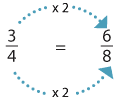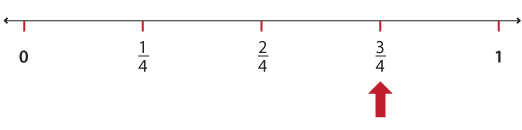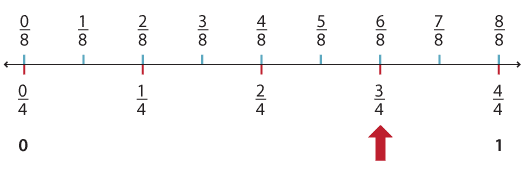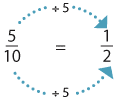### Multiplying and dividing to get equivalent fractions

We have seen that $$\dfrac{3}{4} \text{and} \dfrac{6}{8}$$ are equivalent. You might have noticed that we can multiply the numerator and the denominator of $$\dfrac{3}{4}$$ by the same number to get $$\dfrac{6}{8}$$.Detailed description

This can also be shown on the number line.Detailed description

When we divide the number line into quarters, and then into eighths, we can see that there are 'two times the number of segments' on the number line and each $$\dfrac{1}{8}$$ segment is half the size of a $$\dfrac{1}{4}$$ segment.Detailed description

Dividing the numerator and denominator by the same whole number also results in an equivalent fraction.Detailed description

So we get an equivalent fraction with a related denominator. Fractions with related denominators are easy to work with when adding and subtracting fractions.

Definition: An equivalent fraction to a given fraction can be found by multiplying or dividing the numerator and the denominator of this fraction by the same whole number.